• 2021-06-12 15:37:42

设试验样本数据 x = ( x 1 , x 2 , ⋯   , x n ) x=(x_1,x_2,\cdots,x_n) Y = ( Y 1 , Y 2 , ⋯   , Y n ) Y=(Y_1,Y_2,\cdots,Y_n) ，若拒绝假设
H 0 : a = 0 ( H 1 : a ≠ 0 ) H_0: a=0(H_1:a\not=0)
则诸 Y i Y_i ~ N ( a x + b , σ 2 ) N(ax+b, \sigma^2) i = 1 , 2 , ⋯   , n i=1,2,\cdots,n 。由于 ( a ∧ − a ) n σ 2 ∧ ( n − 2 ) ∑ i = 1 n ( x i − x ‾ ) 2 (\stackrel{\wedge}{a}-a)\sqrt{\frac{n\stackrel{\wedge}{\sigma^2}}{(n-2)\sum\limits_{i=1}^n(x_i-\overline{x})^2}} ~ t ( n − 2 ) t(n-2) ，可将 a ∧ n σ 2 ∧ ( n − 2 ) ∑ i = 1 n ( x i − x ‾ ) 2 \stackrel{\wedge}{a}\sqrt{\frac{n\stackrel{\wedge}{\sigma^2}}{(n-2)\sum\limits_{i=1}^n(x_i-\overline{x})^2}} 作为假设检验量，检验假设 H 0 : a = 0 H_0:a=0
然而，函数linregress函数的返回值所含字段pvalue，就是表示的该统计量值对应检验p值，只需将其与给定的显著水 α \alpha 比较，即可得到检验结果。
当假设 H 0 : a = 0 H_0:a=0 被拒绝时，认为回归效应是显著的。此时，可利用linregress函数的返回值所含的表示样本相关系数 ∑ i = 1 n ( x i − x ‾ ) ( y i − y ‾ ) ∑ i = 1 n ( x i − x ‾ ) 2 ∑ i = 1 n ( y i − y ‾ ) 2 \frac{\sum\limits_{i=1}^n(x_i-\overline{x})(y_i-\overline{y})}{\sqrt{\sum\limits_{i=1}^n(x_i-\overline{x})^2}\sqrt{\sum\limits_{i=1}^n(y_i-\overline{y})^2}} 的字段rvalue来判断回归效应的高低：其绝对值越接近1，意味着回归效应越高。
例1设炼铝厂所产铸模的抗张强度与所用铝的硬度有关。设当铝的硬度为 x x 时，抗张强度 Y Y ~ N ( a x + b , σ 2 ) N(ax+b,\sigma^2) ，其中 a a b b σ 2 \sigma^2 均未知。对于一系列的 x x 值，测得相应的抗张强度如下表
硬度 x : 51 , 53 , 60 , 64 , 68 , 70 , 70 , 72 , 83 , 84 抗张强度 Y : 283 , 293 , 290 , 256 , 288 , 349 , 340 , 354 , 324 , 343 \text{硬度}x: 51,53,60,64,68,70,70,72,83,84\\ \text{抗张强度}Y: 283,293,290,256,288,349,340,354,324,343
希望根据样本数据计算假设 H 0 : a = 0 H_0:a=0 对显著水平 α = 0.05 \alpha=0.05 的检验。
解： 下列代码完成本例计算。

import numpy as np
from scipy.stats import linregress
alpha=0.05
x=np.array([51, 53, 60, 64, 68, 70, 70, 72, 83, 84])
y=np.array([283, 293, 290, 286, 288, 349, 340, 354, 324, 343])
res=linregress(x, y)
print('H0:a=0 is %s'%(res.pvalue>=alpha))
print('correlation coefficient is %.4f'%res.rvalue)


第6行调用linregress函数，返回值为res。第7行利用res的pvalue字段检验回归系数 a = 0 a=0 的假设，第8行显示表示回归效应的res的rvalue字段值。运行程序，输出

H0:a=0 is False
correlation coefficient is 0.6968


表示拒绝假设 H 0 : a = 0 H_0:a=0 ，且样本相关系数值为0.6968，回归效应比较高（超过0.5）。
写博不易，敬请支持：
如果阅读本文于您有所获，敬请点赞、评论、收藏，谢谢大家的支持！
返回《导引》

更多相关内容
• 回归分析中，只包括一个自变量和一个因变量，且二者的关系可用一条直线近似表示，这种回归分析称为一元线性回归分析。如果回归分析中包括两个或两个以上的自变量，且因变量和自变量之间是线性关系，则称为多元线性.....

一、线性回归
1.定义
线性回归是利用数理统计中回归分析，来确定两种或两种以上变量间相互依赖的定量关系的一种统计分析方法，运用十分广泛。其表达形式为y = w’x+e，e为误差服从均值为0的正态分布。
回归分析中，只包括一个自变量和一个因变量，且二者的关系可用一条直线近似表示，这种回归分析称为一元线性回归分析。如果回归分析中包括两个或两个以上的自变量，且因变量和自变量之间是线性关系，则称为多元线性回归分析。
2.操作
（1）准备数据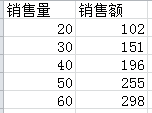本文介绍的是简单的一元线性回归。准备好数据之后，一定要分清楚自变量和因变量。样本数据中很明显销售量属于自变量，而销售额属于因变量。
（2）绘制散点图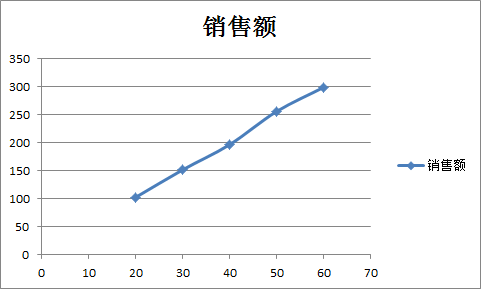绘制散点图的目的是先大致观察一下这两个变量之间是否存在线性相关性，正相关还是负相关。很明显，销售量和销售额具有线性正相关性。
（3）预测结果
当老板问你：“小样儿，如果这个月的销售量是80，根据以往经验看，销售额能达到多少啊？”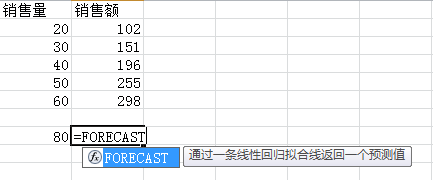FORECAST——这个函数可以用于通过一条线性回归拟合线返回一个预测值。
语法如下：
第一个参数为预测值，第二个参数为因变量的值，第三个参数为自变量的值。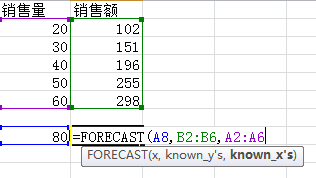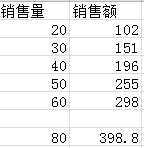二、相关系数
1.定义
相关系数是最早由统计学家卡尔·皮尔逊设计的统计指标，是研究变量之间线性相关程度的量，一般用字母 r 表示。由于研究对象的不同，相关系数有多种定义方式，较为常用的是皮尔逊相关系数。
在实际工作过程中，主要研究两个对象之间是否具有线性相关性，以及相关程度有很大。比如，购买奶粉的顾客是否也会购买尿不湿，这两者之间的关联度有多大？就可以计算出两者的相关系数大小从而得出结论。
2.操作
（1）准备数据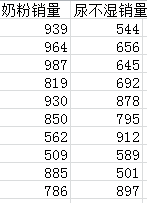（2）绘制散点图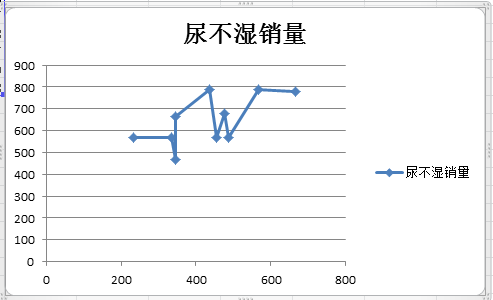**注意：配图有点问题，应该为散点图！！主要是数据是编的，大家理解原理即可！**绘制散点图的目的是初步定性的分析一下，两者是否具有相关性。
（3）相关系数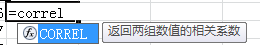CORREL——这个函数可以用来返回两组数值的相关系数。
语法如下：
第一个参数为计算相关系数的对象1的值，第二个参数为计算相关系数的对象2的值。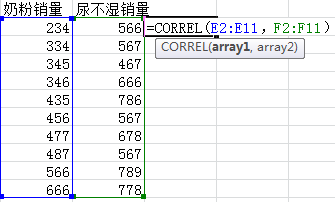结果显示，两者的相关系数为0.648，可以认定两者存在相关性（r>0.6）,一般大于0.6可认为两者属于强相关。进一步说明，买奶粉的顾客中买尿不湿的顾客也很多（反过来也可以解释），可以指导实际零售商进行商品的摆放等操作。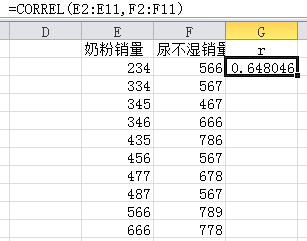展开全文Excel数据分析 Excel数据挖掘
• 利用c#语言实现多元统计分析一元线性回归方程系数计算，可执行。
• 在 Jupyter Notebook 上用 Python 实现的一元线性回归，包括源码和数据，大家可以学习参考使用
• 用 C 语言实现一元线性回归，包括残差平方和，回归平方和，总离差平方和，判定系数，绝对详细注释。
• 回归 最早由英国科学家弗朗西斯高尔顿提出，生物学家，他发现一个现象，虽然有一个趋势“父母高，儿女也高；...一元线性回归 回归分析：用来建立方程模拟两个或者多个变量之间如何关联。 被预测的变量叫做因变量...

# 回归

最早由英国科学家弗朗西斯高尔顿提出，生物学家，他发现一个现象，虽然有一个趋势“父母高，儿女也高；父母矮，儿女也矮”，但给定父母的身高，儿女的身高却趋向于（回归于）全体人口的平均身高，换句话说就是，即使父母都异常高或者异常矮，儿女的身高也会趋近于平均身高，这就是普遍的回归规律。

# 一元线性回归

回归分析：用来建立方程模拟两个或者多个变量之间如何关联。

被预测的变量叫做因变量，被用来进行预测的变量叫自变量，一元线性回归包含一个自变量和一个因变量，通常就是线性关系，若包含两个以上的自变量则称为多元回归分析。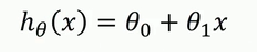求解方程系数：

有方程，有数据集，将数据集带入方程，求解得到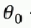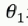，知道了斜率和截距，对于一元回归来说，就相当于构建了一个关于这个数据集的模型。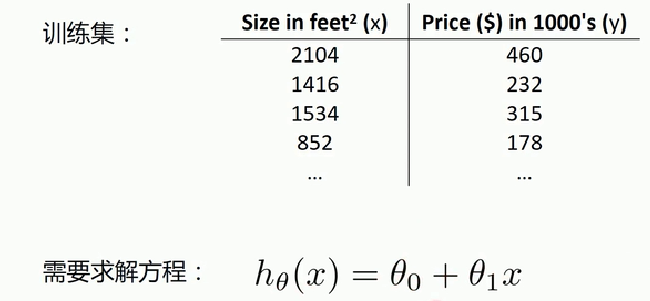# 代价（损失）函数（Cost Function）

运用的是最小二乘法

真实值y,预测值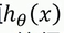,则误差平方为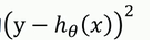找到合适的参数，使得误差平方和：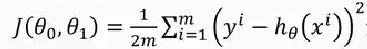最小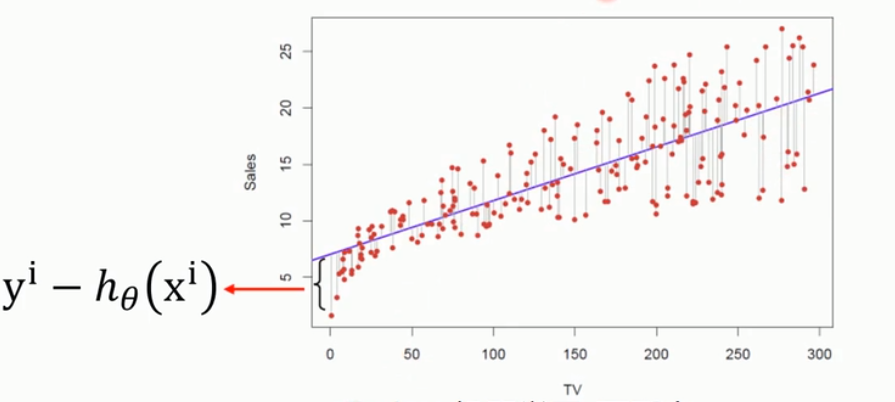代价函数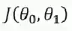等于真实值减去预测值的平方和，再除以样本个数m,这里平方的作用就是让数据为整数，那为啥不用绝对值呢？既然除以的是样本个数，为啥除以的是2呢？

不用绝对值的原因是绝对值对于后面的运算不友好，不好运算，所以就用平方代替，而2m是因为有一个平方，求导之后会多一个2，多除以一个2就是用来和求导后的系数低消的，比较好看，至于为啥要求导，后面会说。

定义代价函数的意义就是为了让代价函数最小，说明拟合的效果越好，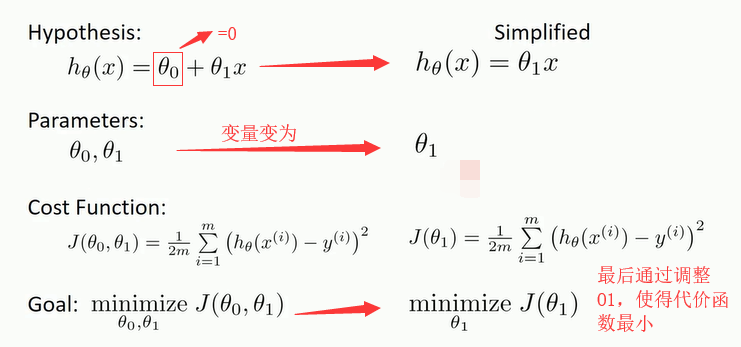例子：

现在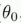为0，必经过原点，我们只调整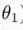使得代价函数最小，左边是有3个样本点，右边横轴为斜率，纵轴为代价函数值，

此时令=1，则曲线为左图，求解代价函数值=（(1-1)^2+(2-2)^+(3-3)^2）/2*3=0，如下图（右）：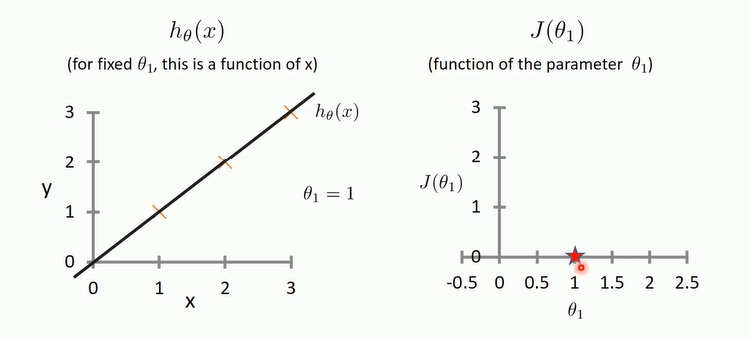=0.5，计算代价函数值=（（1-0.5）^2+（2-1）^2+（3-1.5）^2）/2*3=0.6,如下图：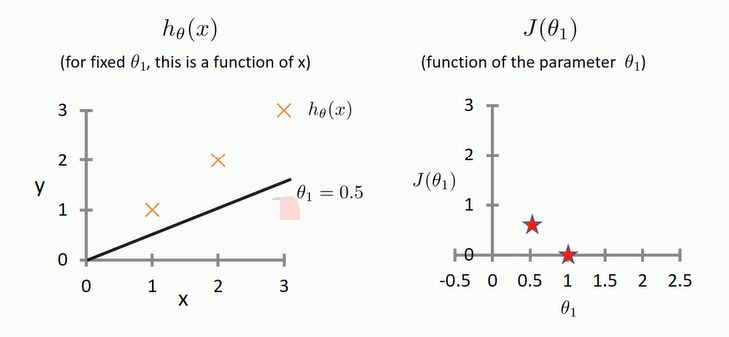=0，计算代价函数值=（（1-0）^2+（2-0）^2+（3-0）^2）/2*3=2.33,如下图：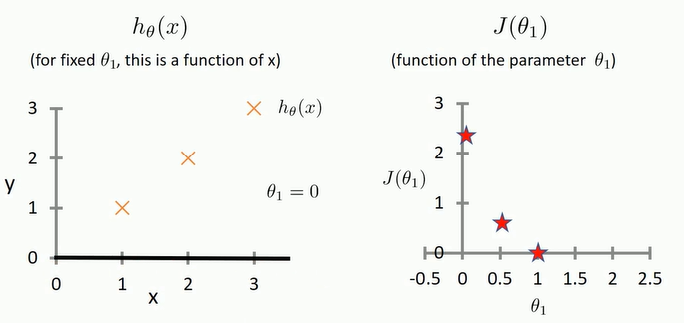取很多的值后会变成：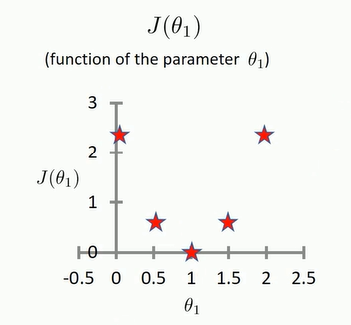可以看出当为1的时候代价函数的值最小，返回去看当=1时的曲线，可以发现确实与数据点拟合的最好的。

# 相关系数

用相关系数去衡量线性相关性的强弱：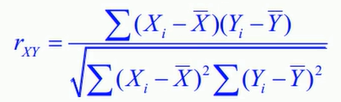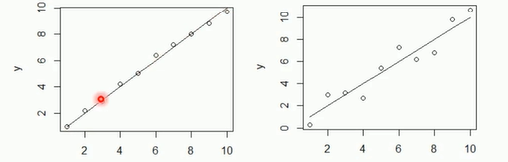计算后，左图相关系数为0.993，右边的图相关系数为0.957，相关系数越接近1，就代表越接近一个线性的关系，越接近于-1就代表越接近于负相关，越接近于零，就代表越不接近一个线性的关系。

# 决定系数

相关系数R用来描述两个变量之间的线性关系，但是决定系数R^2适用范围更广，可用于描述非线性或者有两个以上自变量的相关关系。用它可以评价模型的效果。

总平方和（SST）：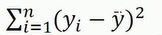回归平方和（SSR）：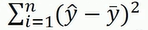残差平方和（SSE）：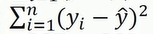三者关系：SST=SSR＋SSE

决定系数：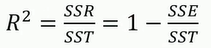展开全文• ## 一元线性回归推导

千次阅读 2022-01-04 10:22:02
一元线性回归的推导

# 一元线性回归系数的推导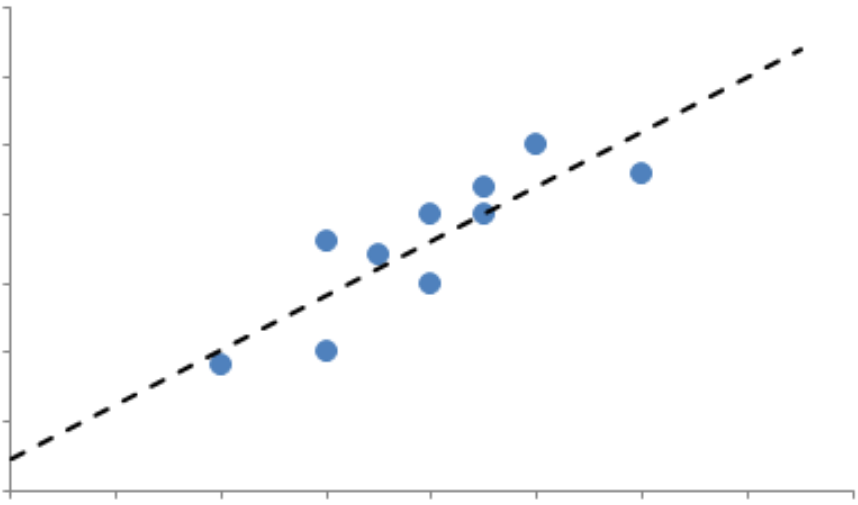假设有n个离散的点 ( x 1 , y 1 ) , ( x 2 , y 2 ) , ⋯   , ( x n , y n ) {(x_1,y_1),(x_2,y_2),\cdots,(x_n,y_n)} ，我们要拟合一条直线 y = m x + b y=mx+b 来寻找 x x y y 的关系。
我们通过最小化平方误差来求拟合直线的系数 m m b b
最小化平方误差squared error
S E L I N E = ∑ i = 1 n ( y i − ( m x i + b ) ) 2 = ∑ i = 1 n y i 2 − 2 m ∑ i = 1 n x i y i − 2 b ∑ i = 1 n y i + 2 m b ∑ i = 1 n x i + m 2 ∑ i = 1 n x i 2 + n b 2 = n y 2 ‾ − 2 m n x y ‾ − 2 b n y ‾ + 2 m b n x ‾ + m 2 n x 2 ‾ + n b 2 \begin{aligned} SE_{LINE}&=\sum_{i=1}^{n}(y_i-(mx_i+b))^2\\ &=\sum_{i=1}^{n}y_i^2-2m\sum_{i=1}^{n}x_iy_i-2b\sum_{i=1}^{n}y_i+2mb\sum_{i=1}^{n}x_i+m^2\sum_{i=1}^{n}x_i^2+nb^2\\ &=n\overline{y^2}-2mn\overline{xy}-2bn\overline{y}+2mbn\overline{x}+m^2n\overline{x^2}+nb^2 \end{aligned}
分别对 m m b b 求偏导可得
{ m x 2 ‾ + b x ‾ = x y ‾ m x ‾ + b = y ‾ \begin{cases} m\overline{x^2}+b\overline{x}=\overline{x y} \\ m\overline{x}+b=\overline{y} \end{cases}
从而有 m = x y ‾ − x ‾ y ‾ x 2 ‾ − x ‾ 2 = C o v ( X , Y ) V a r ( X ) m=\frac{\overline{xy}-\overline{x}\overline{y}}{\overline{x^2}-\overline{x}^2}=\frac{Cov(X,Y)}{Var(X)}
即可得到回归系数。

展开全文回归 机器学习
• python获取留存曲线的幂函数系数计算生命周期LT，中介绍了获取幂函数系数的两种方式，下文对第一种方法【lnx、lny对应的线性拟合，最小二乘法求解系数】进行公式推导。python 最小二乘法
• 以最大积雪深度与灌溉面积之间的关系为例子，包括：基于矩阵运算计算回归系数并添加趋势线，基于多项式拟合计算回归系数并添加趋势线，计算用于检验的主要统计量，借助回归分析程序包计算回归系数并估计检验统计量等...
• 求多幅栅格影像的变化趋势，基于一元线性回归法，得到回归系数
• ## 一元线性回归

千次阅读 2021-12-09 19:08:59
一元线性回归确定变量间的关系模型估计和检验利用回归方程进行预测回归模型的诊断 确定变量间的关系 确定变量间的关系 变量间的关系大体上分为函数关系和相关关系（大致理解：前者是一个变量值的变化完全依赖于另一...回归 r语言
• 变量间的关系分析 变量间的关系有两类，一类是变量间存在完全确定的关系，即函数关系(y=2x+1)，另一类是变量间不存在完全的确定关系，不能用准确的数学公式...需要引入线性相关系数ρ来衡量两变量的相关关系和密切程度
• 2. 计算一元线性回归方程y=ax+b的系数a和b，用两种方法计算：一是公式：；二是用最小二乘法的公式求出最小值点(a,b),使3. 检验回归方程是否有效(用F分布检验)。4. 把散列点(xi，yi)和回归曲线y=ax+b画在一个图上。5....
• 目录一元线性回归一元线性回归建模的大致思路如下：1. 确定因变量与自变量之间的关系1.1 查看变量间有没有相关关系1.2计算相关系数1.3 检验相关系数2.建立模型，并对模型进行估计和检验2.1 回归模型的拟合2.2 计算...r语言 回归 最小二乘法
• 简述线性回归模型是机器学习里面最基础的一种模型，是为了解决回归问题，学习机器学习从线性回归开始最好，网上关于机器学习的概述有很多，这里不再详细说明，本博文主要关注初学者常见的一些问题以及本人的一些思考...
• 一元线性回归分析预测法，是根据自变量x和因变量Y的相关关系，建立X与Y的线性回归方程进行预测的方法。由于市场现象一般是受多种因素的影响，而并不是仅仅受一个因素的影响。所以应用一元线性回归分析预测法，必须对...机器学习 算法 人工智能
• 皮尔逊相关系数的r和一元线性回归分析中的r2的关系区别
• 用SPSS可以做一元线性回归分析，但是当回归的自变量比较多的时候，一个一个的输入会比较麻烦，增加了计算量，本文中描述了如何用matlab语言来实现一元线性回归分析。在matlab中，regress命令是用来做回归的。假如有...
• ## 一元线性回归原理及代码实现

千次阅读 热门讨论 2022-01-03 21:13:03
一元线性回归中的一元指的是只有一个自变量 回归预测的问题是连续值 一元线性回归的目的： 要求解这个方程就需要确定两个参数 怎么求参数？ 介绍一个概念叫做代价函数，也叫损失函数，是衡量真实值与预测值误差的...回归 机器学习
• ## 一元线性回归的详细推导过程

千次阅读 多人点赞 2020-08-30 00:26:17
下面是一元线性回归的详细求解过程。 假设一元线性回归的最佳直线方程为： (1) 对于一个样本点 ，有预测值为： (2) 这个样本点的真值为 ，要想获得最佳拟合方程，就需要使真值 和 预测值 之间的差值最小，为了...
• 一元线性回归分析 一元回归分析的基本概念 回归模型的建立一般包括： （1）通过某事物现，转化为具体问题； （2）确定指标变量，收集整理数据，并构建模型进行参数估计； （3）模型的检验，当模型检验不通过时，需要...回归 数据建模
• 1997年第3期 云南化工 55 计算机应用C语言编程对实验数据进行一元线性回归处理杨继红 尹家元 沈 勇(云南大学化学系 昆明650091)摘 要 用目前最有发展前景的C语言编程处理分析实验及环保数据,其中用函数调用法编程,...
• '所有一元回归函数为Y=' , a , 'x+' , b ) 检验回归效果显著性 1）设 H 0 : b = 0 , H 1 : b ≠ 0 H_0:b=0,H_1:b\not =0 H 0 ​ : b = 0 , H 1 ​ : b  ​ = 0 2）判断 ∣ b ^ ∣ σ ^ S x x 与 t α 2...
•   这篇文章详细推导了一元线性回归方程的参数解，供新手朋友参考。   假定一元线性回归方程的具体形式为 y=a+bx(1) y=a+bx \tag{1} y=a+bx(1) 现在，为确定参数a,ba,ba,b进行了nnn次观测，观测结果为： i123⋯...参数估计 最小二乘法
• Matlab中实际上有多个函数可以实现回归分析的功能，如regress，polyfit，lsqcurvefit等。这里简单总结一下polyfit函数的用法：Matlab中实际上有多个函数可以实现回归分析的功能，如regress，polyfit，lsqcurvefit等...
• 这里写自定义目录标题前言实现原理工具准备1， 线性回归最小二乘参数估计使用python计算最小二乘法的线性回归检验 前言 本次知识来自贾俊平《统计学》第七版，初次学习，如有纰漏，请各位多多包含。诸位之意见笔者会...python
• 一元线性回归方程为，数据样本点为， 要想使这n个样本点落在一元线性回归方程附近，不妨设误差为，使得没一个样本点落在一元线性回归方程上，因此有恒成立，所以回归直线应满足的条件是：实际值与回归估计值之间...最小二乘法 回归...